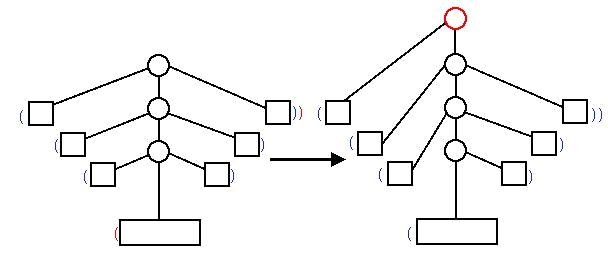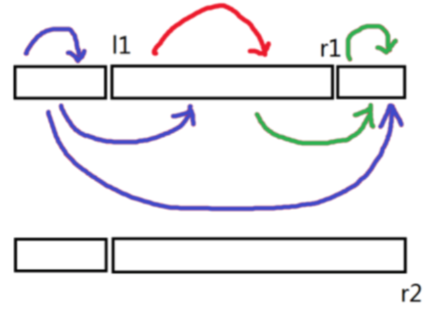# 博客

## [Ynoi2015]Day2题解

2019-10-28 10:48:32 By OldDriverTree

### T1：いまこの時の輝きを（Version1）

Idea：hhf Solution：lxl Std：lxl Data：lxl

https://www.luogu.org/problem/P5071

### T2：どうか、忘れないで（Version1）

Idea：lxl Solution：lxl Std：lxl Data：lxl

https://www.luogu.org/problem/P5072

$2^{len-y}$ 是除了 $x$ 之外的数有这么多个不同的子序列，这些对 $x$ 的贡献没有影响

$2^y-1$ 是所有 $x$ 构成的子序列中有这么多种至少包含一个 $x$ ，有 $1$ 种不包含 $x$

$2^1,2^2…2^{\sqrt n} % p$

### T3：世界で一番幸せな女の子（Version2）

Idea：lxl Solution：ccz Std：lxl Data：lxl

https://www.luogu.org/problem/P5073

cur -> L()为cur节点内部的左端最大前缀和的一个分段函数

cur -> R()为cur节点内部的右端最大后缀和的一个分段函数

cur -> MID()为cur节点内部的最大子段和的一个分段函数

cur -> L(x)代表cur节点整体被加了$x$后其最大前缀和

cur -> R(x)代表cur节点整体被加了$x$后其最大后缀和

cur -> MID(x)代表cur节点整体被加了$x$后其最大子段和

cur -> L(x) = max( cur -> left -> L(x) , cur -> right -> L(x) + cur -> left -> sum );

cur -> R(x) = max( cur -> left -> R(x) + cur -> right -> sum , cur -> right -> R(x) );

cur -> MID(x) = max( cur -> left -> MID(x) , cur -> right -> MID(x) , cur -> left -> R(x) + cur -> right -> L(x) );## [Ynoi2015]Day1题解

2019-10-28 09:58:08 By OldDriverTree

### T1：ただいま帰りました（Version3）

Idea：lxl Solution：lxl Std：lxl Data：lxl

https://www.luogu.org/problem/P5068

### T2：いずれその陽は落ちるとしても（Version?）

Idea：ccz Solution：ccz Std：ccz Data：ccz

https://www.luogu.org/problem/P5069

### T3：たとえ未来が見えなくても（Version3）

Idea：lxl Solution：lxl Std：lxl Data：lxl

https://www.luogu.org/problem/P5070## [Ynoi2014]Day2题解

2019-10-27 11:52:44 By OldDriverTree

### T1：帰らぬ者と、待ち続けた者たち（Version?）

Idea：kcz Solution：kcz/lca Std：kcz/lca Data：kcz

https://www.luogu.org/problem/P5065

$k$取$\sqrt n$时，

### T2：誰も彼もが、正義の名のもとに（Version5）

Idea：lxl Solution：lxl Std：lxl Data：lxl

https://www.luogu.org/problem/P5066

### T3：消えない過去、消えていく未来（Version?）

Idea：mcfx Solution：mcfx Std：mcfx Data：mcfx

https://www.luogu.org/problem/P5067

+ 和 - 节点由于运算优先级相同，可以一起处理。

1.加入一个括号；

2.删除一对括号；

3.加入或删除一个其他节点。## [Ynoi2014]Day1题解

2019-10-19 02:35:57 By OldDriverTree

### T1：太陽の傾いたこの世界で（Version1）

Idea：ccz Solution：ccz Std：ccz Data：ccz

https://www.luogu.org/problem/P5062

### T2：空の上の森の中の（Version1）

Idea：zcy Solution：lxl Std：lxl Data：lxl

https://www.luogu.org/problem/P5063

1.区间加

2.单点修改

3.区间rank

$O( m \sqrt n + m \sqrt {n/2} + m \sqrt {n/4} + ... )$ = $O( m \sqrt n )$

### T3：この戦いが終わったら（Version3）

https://www.luogu.org/problem/P5064## [Ynoi2018]Day2题解

2019-09-16 12:24:16 By OldDriverTree

### T1：そらのおとしもの（Version2）

Idea：lxl Solution：lxl Std：lxl Data：lxl

https://www.luogu.org/problem/P5397

http://www.51nod.com/Challenge/Problem.html#problemId=2046

"弑尽破净的第四分块" （妈呀这名字好中二啊（谁叫我要用日本轻小说中的东西命名真是作死））

$O( n \sqrt n + m \sqrt n )$

x取遍所有值，y只有所有大的值，总$O( \sqrt n )$个### T2：ゴシック（Version2）

Idea：lxl Solution：lxl Std：lxl Data：lxl

https://www.luogu.org/problem/P5398

https://cometoj.com/problem/1072

"点缀光辉的第十四分块" （妈呀这名字好中二啊（谁叫我要用日本轻小说中的东西命名真是作死））

$[l1,r1]$区间的答案为红色部分的贡献，$[l1,r2]$区间的答案为红色部分加上绿色部分的贡献，$r2$的前缀贡献为所有的贡献，$r1$的前缀贡献为红色的贡献与前两个蓝色的贡献$[l,r]$中$x$的倍数个数：每次前缀加入一个数$a_i$的时候，我们把$a_i$的约数位置所对应的数组$pre1$的位置都++

$[l,r]$中$x$的约数个数：这里考虑对$x$的约数进行一次根号分治### T3：駄作（Version1）

Idea：lxl Solution：ccz Std：ccz Data：ccz

https://www.luogu.org/problem/P5399

https://loj.ac/problem/6612

"？？？？的第七分块" （不中二了）

1.每个块是树

2.每个块中有两个特殊的点，称为端点 1 和端点 2。

3.不同块的边集不相交

4.一个块中的顶点，除端点外，其余顶点不在其它块中出现

5.如果一个顶点在多个块中出现，那么它一定是某一个块的端点 2，同时是其余包含这个顶点的块的端点 1

6.如果把所有块的端点作为点，每块的端点 1 和端点 2 连有向边，则得到一棵有根树